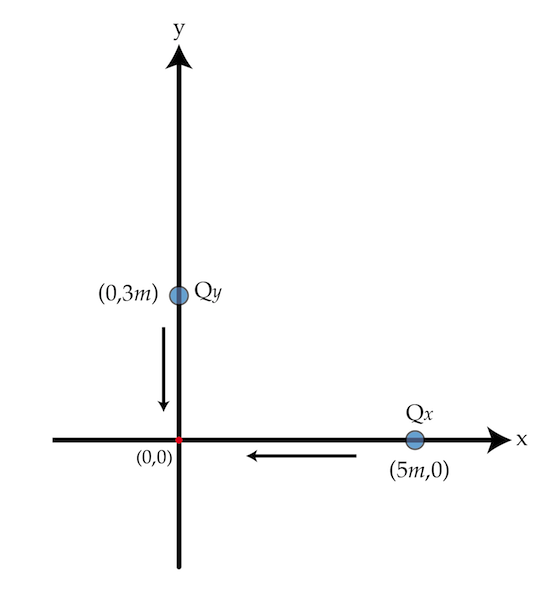Two 1-g beads with charges $Q_{x}=2~\mu\mbox{C}$ and $Q_{y}=-3~\mu\mbox{C}$ slide on (without friction) two perpendicular wires ($x$ and $y$ axes). The charges are initially located at $(5~\mbox{m},0)$ and $(0,3~\mbox{m})$ and released from rest.What is the distance in meters between the charges when $Q_{y}$ crosses the origin $(0,0)$?

Details and assumptions

$k=\frac{1}{4\pi \epsilon_{0}}=9 \times 10^{9} \mbox{N m}^{2}/\mbox{C}^{2}$

×